Learning Library

# Single Digit Multiplication Practice for 2nd Grade

Is your child familiar with multiplying single digit numbers together? Go over multiplication strategies with your child, and encourage her to do the worksheets again and beat her last time!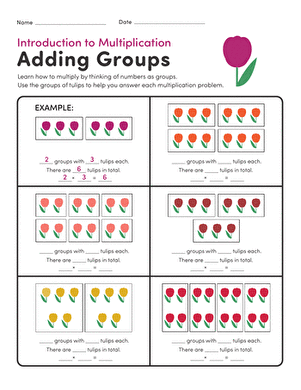### Intro to Multiplication: Adding Groups

Start your child on multiplication by practicing adding groups of a certain number using flowers to help.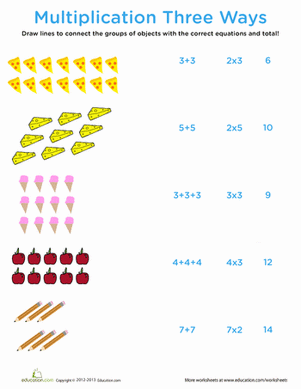### Intro to Multiplication: Multiplication Three Ways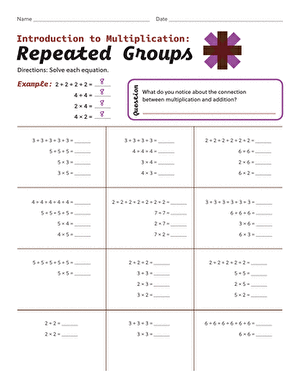### Intro to Multiplication: Repeated Groups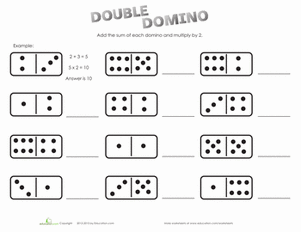### Intro to Multiplication: Multiplying by 2

Turn domino play into multiplication practice with this worksheet that helps kids learn how to multiply by 2.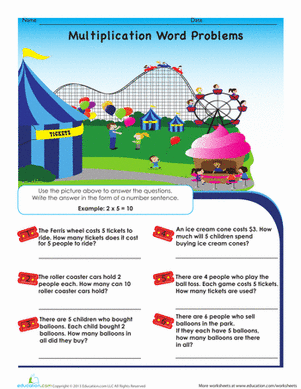### Intro to Multiplication: Roller Coaster Word Problems

Practice early multiplication skills with carnival-themed word problems packed with roller coasters, clowns, and cotton candy.### Multiply by 0

This worksheet is a great way to get your kid started on multiplication by helping him multiply by 0.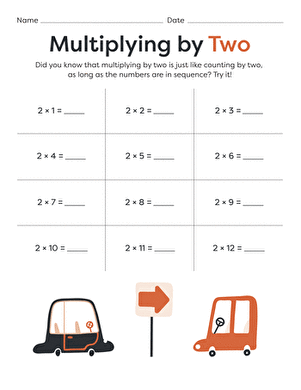### Multiplying by 2

Practice multiplying by 2 the easy way: just pretend you're counting by twos!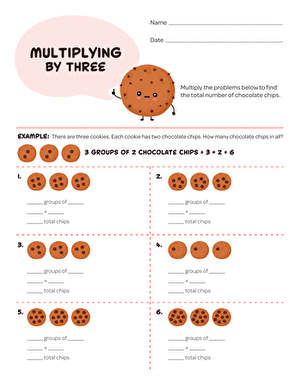### Multiplying by 3

Practice multiplying by 3 with this sweet, cookie-themed worksheet. Multiplication is a lot more fun when ice cream is involved!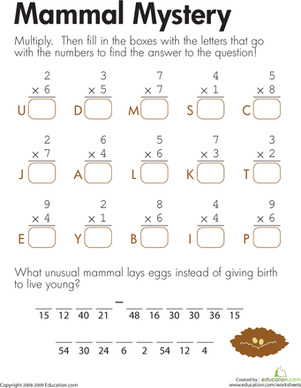### Multiplication: Mammal Mystery

Kids solve multiplication problems to crack the code and find the egg-laying mystery mammal on this second grade math worksheet.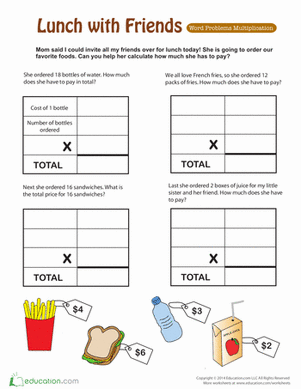### Lunch with Friends: Multiplying Money

Multiplying money word problems let your child practice his multiplication while figuring out how much lunch costs. A good way to learn the concept of money!

Create new collection

0

### New Collection>

0 items

What could we do to improve Education.com?